•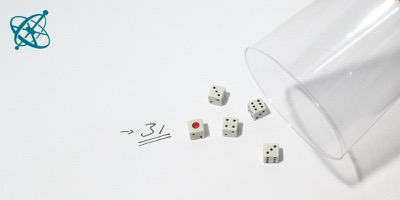Link the values on the dice…

•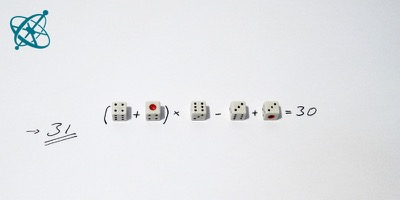…by arithmetic operations to come…

•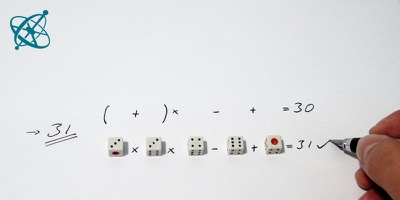…as close to the target number as possible.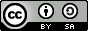www.sciensation.org | Ciênsação hands-on experiments are published as Open Educational resources under a Creative Commons Attribution-ShareAlike 4.0 International License.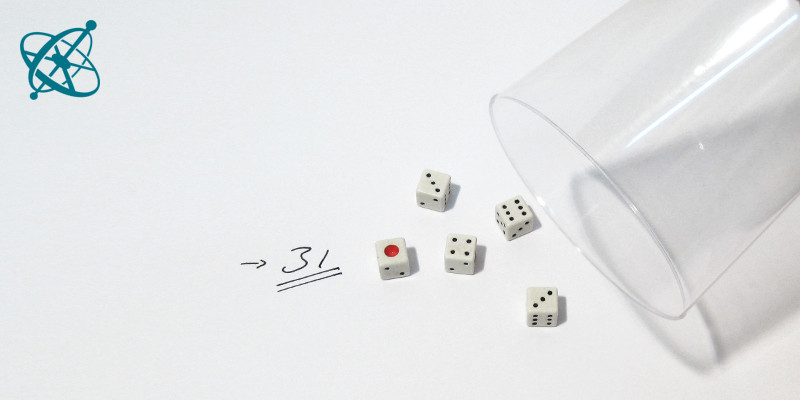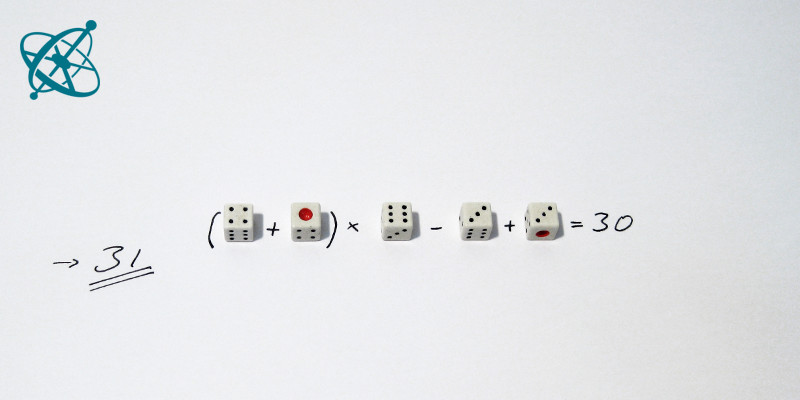Maths Game
age: 7 – 16

# Arithmagic

A simple dice game to exercise mental arithmetic, e.g. if you have to stand in for a colleague in a cover lesson.

Learning objective

Exercising mental arithmetic.

Preparation

Ask your students to form teams of two and then group three teams together. Hand each group 5 dice. Then you choose a random number between 1 and 50 as target number and let your students play a round.

Since always two teams compete in getting the result closest to the target number, they should discuss in a secretive lower voice and check carefully each others' arithmetic. Repeat as often as you like.

Rules of Arithmagic:

1. Your teacher selects a random number between 1 and 50 as target number.

2. In each group, one team takes on the role of croupier. This team rolls 5 dice for the other teams, controls the time – one minute – and that nobody is cheating.

3.Within this time, the two teams try to combine all numbers on the dice in any order by arithmetic operations (summation, subtraction, multiplication, division) to get as close to the target number as possible.

4. If a team's calculation is correct, it writes down the absolute difference of its result to the target number as points. In case of an error in their calculation or if they fail to come to a result, they write down half of the target number as their points.

5. Then the next team takes the role of croupier and the steps 2 to 5 are repeated until each team was once croupier and played twice.

6. Each team sums up their points and the team with the lowest number of points wins.

Findings

As simple as the game may seem, as challenging and complex it can become as you add more dice.

As an example, assume a target number of 31 and dice showing 4, 6, 3, 1, and 3. Two possible options are:

(4 + 1) · 6 + 3 - 3 = 30 ➥ 31-30 = 1 point

3 · 3 · 4 - 6 + 1 = 31 ➥ 0 points

You can easily simplify the game for younger students with less dice and lower target numbers (e.g. to avoid multiplication and division), or make it more challenging by adding dice.

Related Experiments
Tags